Students can download Maths Chapter 5 Coordinate Geometry Ex 5.4 Questions and Answers, Notes, Samacheer Kalvi 9th Maths Guide Pdf helps you to revise the complete Tamilnadu State Board New Syllabus, helps students complete homework assignments and to score high marks in board exams.

## Tamilnadu Samacheer Kalvi 9th Maths Solutions Chapter 5 Coordinate Geometry Ex 5.4

Question 1.
Find the coordinates of the point which divides the line segment joining the points A (4,-3) and B (9,7) in the ratio 3 : 2.
Solution:
A line divides internally in the ratio m : n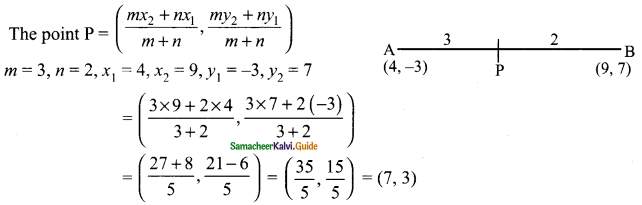Question 2.
In what ratio does the point P(2, -5) divide the line segment joining A(-3, 5) and B(4, -9).
Solution:
A line divides internally in the ratio m : n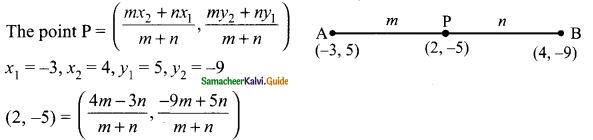$$\frac{4m-3n}{m+n}$$
= 2
4m – 3n = 2m + 2n
4m – 2m = 3n + 2n
2m = 5n
$$\frac{m}{n}$$ = $$\frac{5}{2}$$
m : n = 5 : 2
The ratio is 5 : 2.
and
$$\frac{-9m+5n}{m+n}$$
= -5
-9m + 5 n = -5(m + n)
-9m + 5 n = -5m – 5n
-9m + 5 m = -5n – 5n
-4m = -10
$$\frac{m}{n}$$ = $$\frac{10}{4}$$ ⇒ $$\frac{m}{n}$$ = $$\frac{5}{2}$$
m : n = 5 : 2Question 3.
Find the coordinates of a point P on the line segment joining A(1, 2) and B(6, 7) in such a way that AP = $$\frac{2}{5}$$ AB.
Solution:
Let the point A (1, 2) and B (6, 7)
AP = $$\frac{2}{5}$$ AB
$$\frac{AP}{PB}$$ = $$\frac{2}{5}$$
∴ AP = 2; PB = 5 – 2 = 3
A line divides internally in the ratio m : n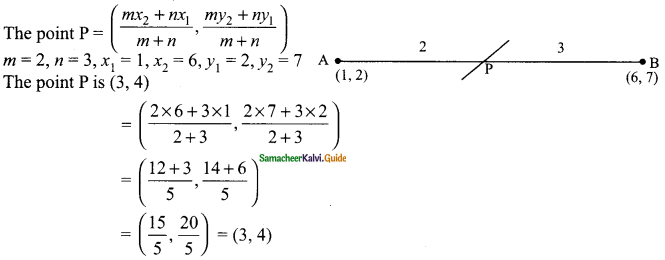Question 4.
Find the coordinates of the points of trisection of the line segment joining the points A (-5, 6) and B (4, -3).
Solution:
Let P and Q be the point of trisection
so that AP = PB = QB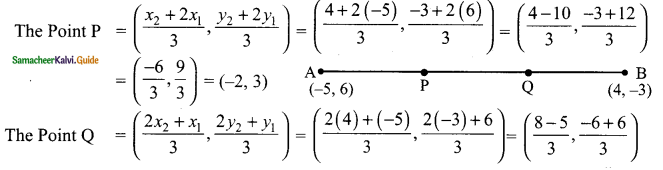($$\frac{3}{3}$$, $$\frac{0}{3}$$) = (1, 0)
The Point P is (-2, 3), The Point Q is (1, 0)Question 5.
The line segment joining A(6, 3) and B(-1, -4) is doubled in length by adding half of AB to each end. Find the coordinates of the new end points.
Solution: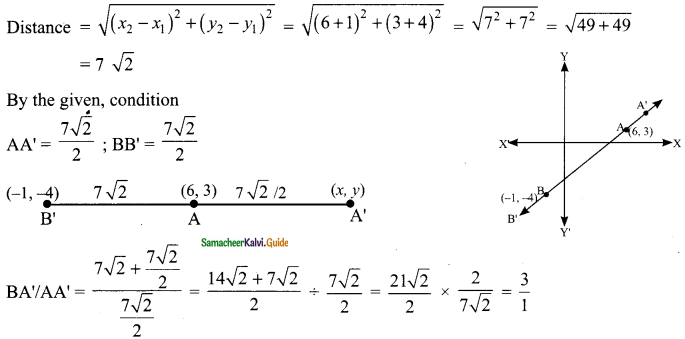m : n = 3 : 1
A line divides externally in the ratio m : n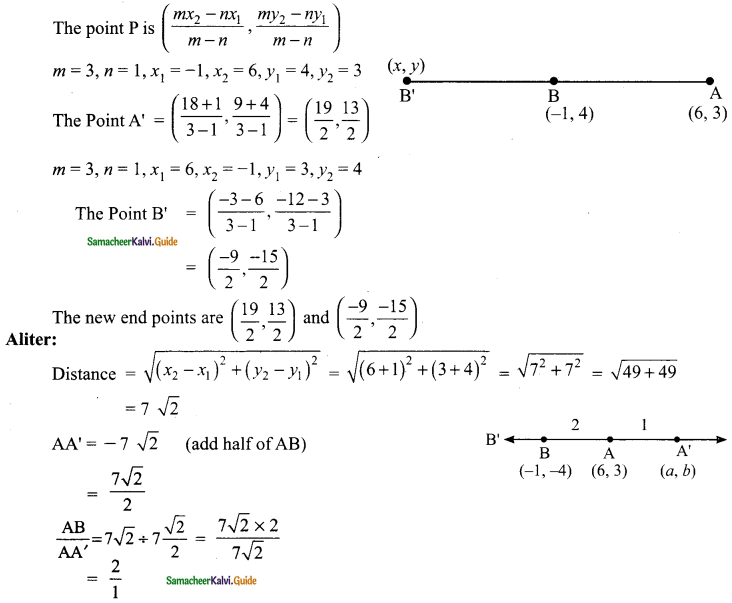∴ BA’ divides in the ratio 2 : 1
A line divides internally in the ratio m : n the point is $$\frac{mx_{2}+nx_{1}}{m+n}, \frac{my_{2}+ny_{1}}{m+n}$$
Let the point A’ be (a, b)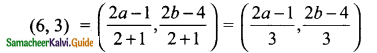$$\frac{2a-1}{3}$$ = 6
2a – 1 = 18
2a = 19
a = $$\frac{19}{2}$$
and
$$\frac{2b-4}{3}$$ = 3
2b – 4 = 9
2b = 13
b = $$\frac{13}{2}$$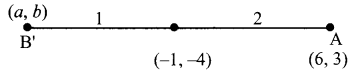The point A’ is ($$\frac{19}{2}$$, $$\frac{13}{2}$$)
To find B’
Let B’ be (a, b)
AB = 7√2
BB’ = $$\frac{1}{2}$$ × 7√2 = $$\frac{7√2}{2}$$
$$\frac{AB}{BB’}$$ = 7√2 ÷ $$\frac{7√2}{2}$$ = $$\frac{7√2×2}{7√2}$$ = 2
AB’ divides in the ratio 2 : 1
(-1, -4) = $$\frac{2a+6}{3}$$, $$\frac{2b+3}{3}$$
$$\frac{2a+6}{3}$$ = -1
2a + 6 = -3
2a = -3 – 6
2a = -9
a = –$$\frac{9}{2}$$
and
$$\frac{2b+3}{3}$$ = -4
2b + 3 = -12
2b = -12 – 3
2b = -15
b = –$$\frac{15}{2}$$ = -1
The point B’ is (-$$\frac{9}{2}$$, –$$\frac{15}{2}$$)Question 6.
Using section formula, show that the points A (7, -5), B (9, -3) and C (13, 1) are collinear.
Solution:
If three points are collinear, then one of the points divide the line segment joining the other points in the ratio r : 1. If P is between A and B and $$\frac{AP}{PB}$$ = r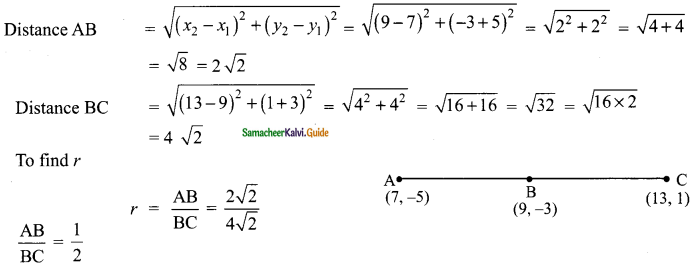The line divides in the ratio 1 : 2
A line divides internally in the ratio m : n
The point P = ($$\frac{mx_{2}+nx_{1}}{m+n}$$, $$\frac{my_{2}+ny_{1}}{m+n}$$)
m = 1, n = 2, x1 = 7, x2 = 13, y1 = – 5, y2 = 1
By the given equation,
The Point B = ($$\frac{13+14}{3}$$, $$\frac{1-10}{3}$$)
= ($$\frac{27}{3}$$, $$\frac{-9}{3}$$)
= (9, -3)
∴ The three points A, B and C are collinear.Question 7.
A line segment AB is increased along its length by 25% by producing it to C on the side of B. If A and B have the coordinates (-2, -3) and (2, 1) respectively, then find the coordinates of C.
Solution:
Let the point C be (a, b)The ratio is 4 : 1 (m : n)
A line divides internally in the ratio m : n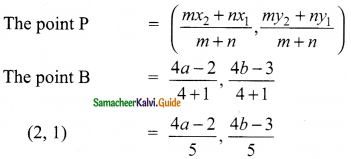$$\frac{4a-2}{5}$$ = 2
4a – 2 = 10
4a = 12
a = $$\frac{12}{4}$$ = 3
and
$$\frac{4b-3}{5}$$ = 1
4b – 3 = 5
4b = 8
b = $$\frac{8}{4}$$ = 2
The co-ordinate of C is (3, 2)